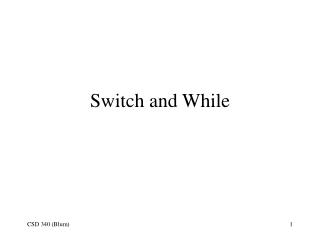DownloadDownload PresentationSwitch and While

# Switch and While

Télécharger la présentation## Switch and While

- - - - - - - - - - - - - - - - - - - - - - - - - - - E N D - - - - - - - - - - - - - - - - - - - - - - - - - - -
##### Presentation Transcript

1. Switch and While

2. From if to switch

3. Concentric tables: alternating colors (I)

4. Concentric tables: alternating colors (II)

5. Concentric tables: alternating colors (III)

6. if(i % 2 == 0) { Color= "FFCC99"; } else { Color="99CCFF"; } • The alternating colors resulted from dividing the counter i by 2 and asking if the remainder is 0. (It can only be 0 or 1.) If the remainder is 0, the Color variable is assigned one value, otherwise it is assigned another.

7. Three Colors

8. Three Color Code (version 1)

9. Switching to a better statement • In a situation in which you find yourself asking almost the same question over and over • Is the expression equal to Value1? • Is the expression equal to Value2? • Is the expression equal to Value3? • Etc. • There is a special statement to replace the sequence of ifs It is called a switch. All of the various conditions are called cases. • See page 84 in Beginning JavaScript (Paul Wilton)

10. Same result with switch

11. Three Color Code (version 1)

12. Expression upon which cases are based Beginning of code to be executed if expression had the value of 0. switch(i % 3) { case 0: Color = "FFCC99"; break; case 1: Color = "99CCFF"; break; case 2: Color = "FFCCCC"; break; } If you are done executing code associated with the condition of the expression having a value of 0, then leave the switch structure.

13. Give me a break! • A switch has a strange behavior. If the expression is equal to 1, the program executes the case 1 code. However, it will continue to execute the code for the following cases unless you explicitly put in that break code that tells it to exit the switch structure.

14. Missing break.

15. It loses the bluish color (case 1).

16. The craps game is a situation in which one might find this “fall through” behavior useful. There are several cases that yield the same effect. switch(sum) { case 2: alert(“Snake eyes”); break; case 3: alert(“Sorry you lose.”); break; case 4: case 5: case 6: alert(“The point is ”+sum+ “ Roll again.”); break; … }

17. If versus Switch • The more “cases” there are, the more convenient it is to use a “switch”. • But use “if” when the condition is an inequality such as less than or greater than. • Also use “if” when the condition involves comparing an expression to a variable as opposed to a set value. • E.g. you cannot use a switch statement in the second roll of craps because of of the cases involves the sum equaling the point which is a variable determined by the first roll.

18. Ripe for switch: Six cases

19. Six cases (code)

20. While loop • A variation on the for-loop repetitive structure is the while loop. • The for loop has a built in counting element and is more suitable in situations in which one knows when starting the loop how many iterations there will be. • The while loop is more suitable in situations in which one does not know the number of iterations at the start of the loop.

21. While loop example • If you were testing your craps program, you might have noticed that you never seem to roll a snake eyes when you want to. • You might ask the question: How many rolls does it take to roll snake eyes for the first time? • It’s possible you could roll it right off, but it’s possible it could take hundreds of rolls.

22. Waiting for Snake Eyes (Code I)

23. Waiting for Snake Eyes (Code II)

24. Waiting for Snake Eyes (Browser I)

25. Waiting for Snake Eyes (Browser II)

26. while(sum!=2) { diceThrow1 = (Math.floor(Math.random() * 6) + 1); diceThrow2 = (Math.floor(Math.random() * 6) + 1); sum=diceThrow1+diceThrow2; //alert(sum); TimesRolled++; } • This says that so long as the the sum variable is not equal to 2 that the program will continually simulate the roll of two dice, add their results and increment a counter. • The variable sum can be started off at any value other than 2 so that we get into the loop in the first place. • The variable TimesRolled starts off at zero. It counts the number of times we rolled the dice and before the loop we rolled them zero times. • Note that any counting must be done inside the the while loop’s curly brackets.

27. Reference • Beginning JavaScript (Paul Wilton)